2019-12-29
###### What are frequencies in images?
2019-12-31
• cv2 and mss basic
• Image Fourier Transform – Introduction and Basics
• Image Fourier Transform – Fourier Transform in Python
• Image Fourier Transform – Low-Pass Filter

## Introduction

Do you know Fourier Transform on signal processing? Fourier Transform is a mathematical method to analyze frequency components in one-dimensional signals, such as sound or radio waves. By applying Fourier Transform on such signal, which is time-domain information, we can know, for example, how much 3000 Hz component is included in the signal.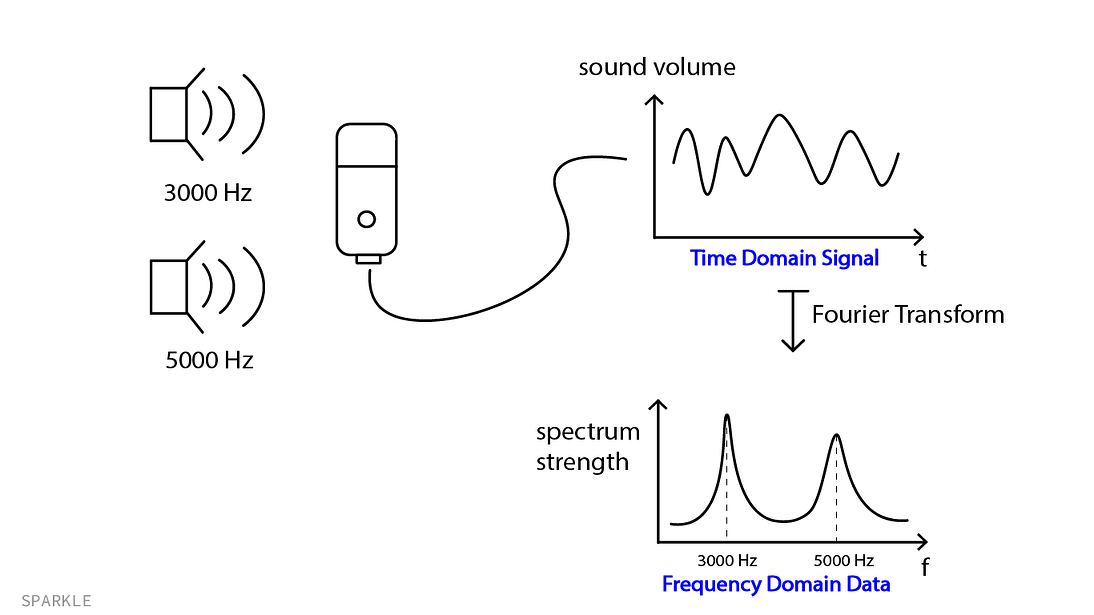This is an example of a 1-dimensional Fourier Transform on sound information recorded by a mic. If Fourier Transform is applied to the record (time-domain info), we can get another graph, which has ‘frequency’ as the horizontal axis. This graph has two peaks at 3000 Hz and 5000 Hz.

But it also can be applied to 2-dimensional information, such as images. This type of Fourier Transform is called 2-D Fourier Transform. In the next few tutorials, I’m going to show you how to use 2-D Fourier on images and why it is so popular in computer vision.

## What is 2-D Fourier Transform?

This is a type of Fourier Transform that takes 2-dimensional data (2-D numpy array) as input and returns another 2-dimensional data. We usually use this 2-D Fourier Transform on images.

Here is an example of applying Fourier Transform on a grayscale image: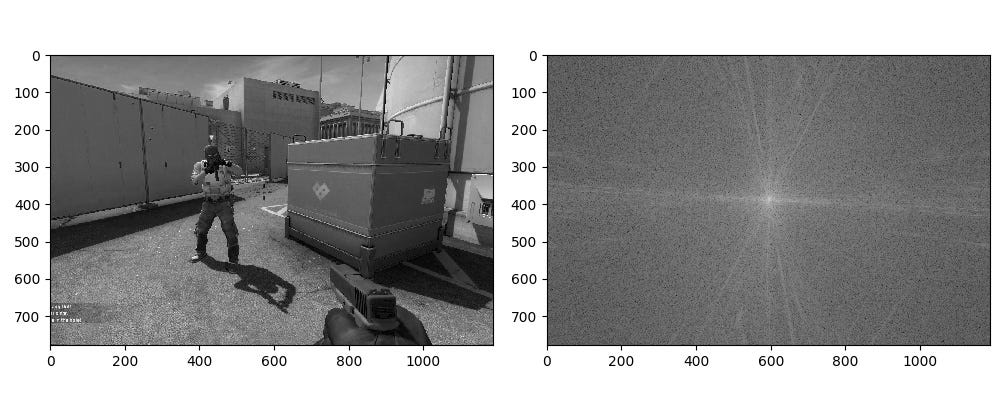Left: original gray image, Right: Fourier Transform of this image. The image size stays the same: (778, 1188)

Each element of the output is a complex number. So we usually take the absolute values of the output such that it can be displayed as an image.

There is also the inverse of Fourier Transform (IFT), which takes a frequency domain image as input and then restores the original image. We can make use of this inverse transform to apply some interesting techniques to images, such as Low Pass Filter or motion estimation of the camera.

## How to interpret the frequency domain image?

You can see that the resulting image of the example above has the brightest pixels (larger values) at the center and outer size pixels relatively less bright (smaller values).

The output frequency domain image tells us how much each frequency component is included in the original image. Pixels near the center represent lower frequency components, and outer side pixels represent higher frequency components. Thus, if pixels near the center are brighter than other outer side, this means that the original image is composed of lower frequency components more than higher frequency components.

Here, a lower frequency component of an image appears in the original image as the rough shape of large objects. Conversely, a higher frequency component can add some details into the image, such as the texture of the ground in the example image above. Remember that small objects and edges of objects are mainly made of higher frequencies.

## Applications of Fourier Transform

### 1 Low Pass Filter

So if we remove higher frequency components from the frequency domain image and then apply Inverse Fourier Transform on it, we can get a blurred image. This is the principle of the Image Low Pass Filter.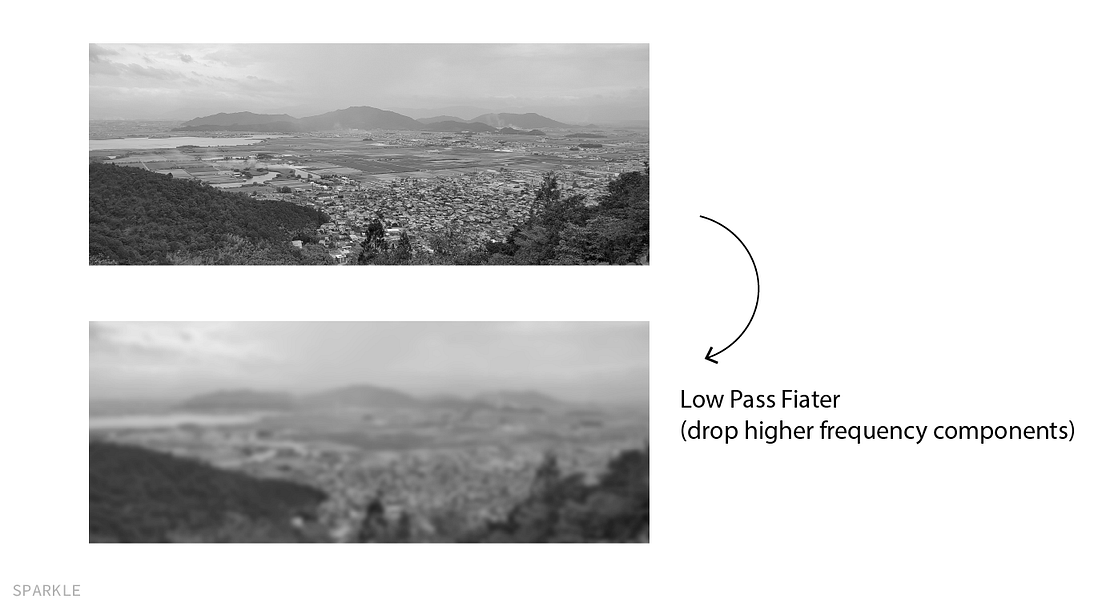If an image is passed to a Low Pass Filter, the result will lack some details (small objects )and edges will be blurred. This is a type of image compression.

## 2 Shift Estimation

Remember that the output of Fourier Transform is an image with complex numbers and we calculated the absolute values of them such that it can be shown as a visible image. But can we make use of the complex values and extract some useful information out of them? The answer is Yes!

Let’s say that there are two images that look similar but are slightly shifted. We can estimate how much and in what direction the images are shifted by comparing their Fourier Transforms.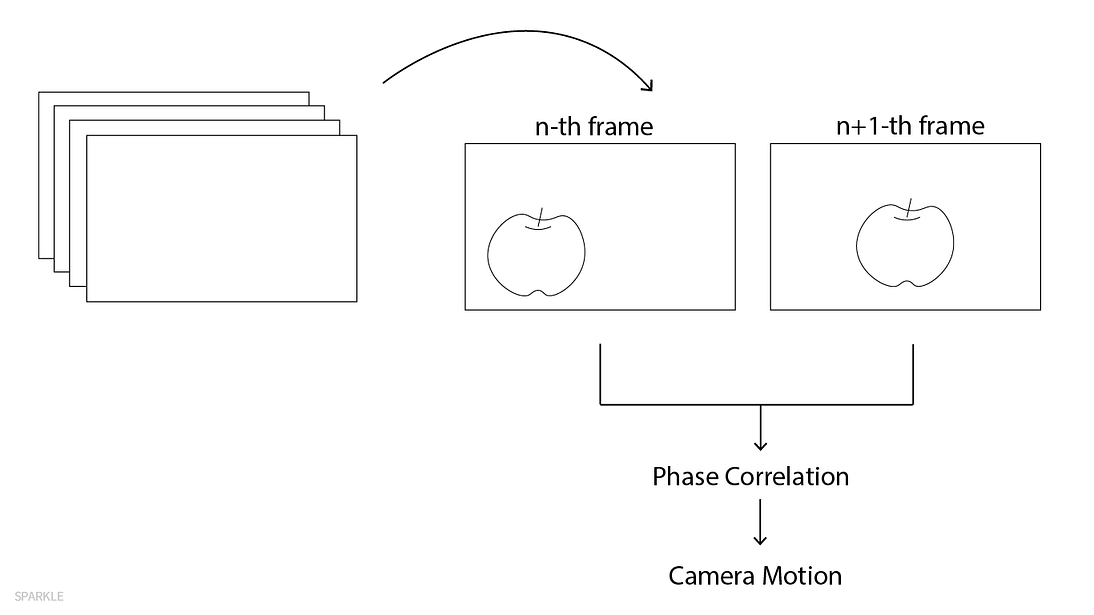By doing some calculations on the two results of Fourier Transform, we can estimate how much the next frame is shifted from the previous frame.

This technique can be used to do video stabilization on videos that are already taken. Once we knew how much two consecutive frames of a video are shifted, we can artificially shift the second frame such that they are not shifted anymore.

## Image Fourier Transform with cv2

In this section, I’m going to show you how to actually apply Fourier Transform with cv2 or numpy. After that, I show you which module is suitable for this purpose. We first load an image and pick up one color channel, on which we apply Fourier Transform. The transform is done simply with `cv2.dft()` function. Here, “dft” means “discrete fourier transform”, since an image is a collection of discrete values, not continuous ones.

```img = cv2.imread('terrorist.jpg') # load an image
img = img[:,:,2] # blue channel
plt.imshow(img, cmap='gray')
```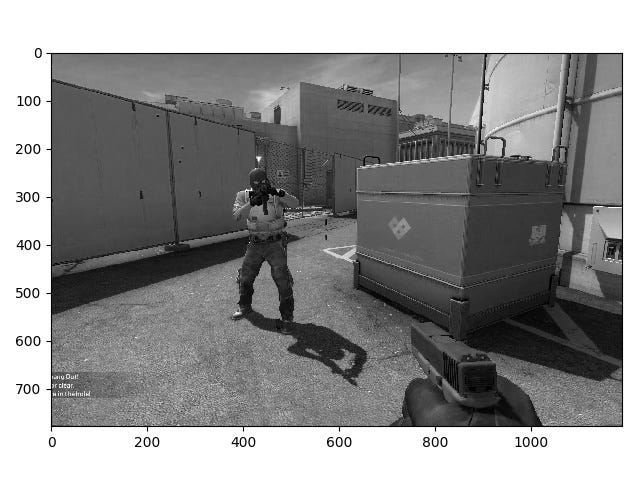This is a grayscale image, which has a shape (778, 1183)
```f = cv2.dft(np.float32(img), flags=cv2.DFT_COMPLEX_OUTPUT)
f_shift = np.fft.fftshift(f)
f_complex = f_shift[:,:,0] + 1j*f_shift[:,:,1]
f_abs = np.abs(f_complex) + 1 # lie between 1 and 1e6
f_bounded = 20 * np.log(f_abs)
f_img = 255 * f_bounded / np.max(f_bounded)
f_img = f_img.astype(np.uint8)
```

The output of `cv2.dft()` function is a 3-dimensional numpy array of shape (778, 1183, 2). Since, in mathematics, the output of 2-D Fourier Transform is a 2-dimensional complex array, the first and second channels of `f` are the real part and imaginary part respectively. `f_complex` is, thus, the complex version of `f`.

In the following few lines, we convert the complex numpy array `f_complex` to the one which can be shown as an image.
At the 5th line, we take the logarithm of the absolute value of `f_complex`, because `f_abs` has a tremendously wide range. The last 3 lines make sure that all elements of `f_img` lie between 0 and 255.

Do not forget to add 1 to `f_abs`, since the argument to the logarithm function should be greater than or equal to 1.0. Also note that the data type of `f_img` is 8-bit unsigned-int, because we want to display it as an image.

Brighter pixels correspond to larger values. Thus, this result means that the original image contained lower frequency components more than higher frequency components.

### Image Fourier Transform with NumPy

You can also use numpy’s `np.fft.fft2()` function instead of cv2. However, it is a little bit slower than `cv2.dft()`.

```f = np.fft.fft2(img)
f_shift = np.fft.fftshift(f)
f_complex = f_shift
```

Since the returned value of np.fft.fft2() is already a complex numpy array, you don’t need to convert it explicitly.
The result is the save as above.

### Benchmarks

I did a benchmark test to see which transform function is faster. The result shows that cv2.fft() is always faster than np.fft.fft2() regardless of the array size. The difference in their performance gets exponentially larger as the array size increases.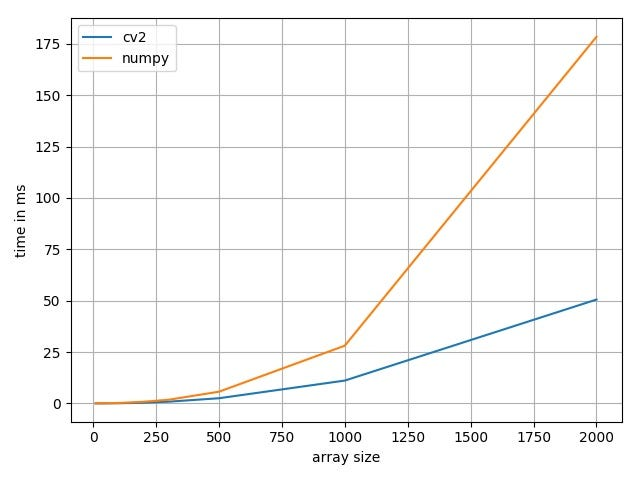The time needed to apply Fourier Transform on several sizes of images.

Both transform function is quite easy to use. However, if we want to use Fourier Transform in real-time speed, we should use `cv2.fft()` function rather than `np.fft.fft2()`.

## Low-pass filter

In this section, we actually use the results of transform to apply a low-pass filter on images. A low-pass filter is a technique used in computer vision to get a blurred image or to store an image with less space.

A low-pass filter can be applied only on the Fourier Transform of an image (frequency-domain image), rather than the original image (spacial-domain image). After applying a low-pass filter to it, then the filtered frequency-domain image is restored into a pixel image, which is a blurred version of the original image.

Actually, a low-pass filter is just a gray-scale image, whose values are higher near the center, and close to zero outside. Therefore, low-pass filters usually look like the following image. This is one of the most popular filters called “Hamming window (wiki)”.

We then multiply this gray-scale image by the Fourier Transform of the original image. What we get is another frequency-domain image, whose values outer side are squashed to zero. This means that higher frequency components are removed, and lower frequency components remain unchanged.
The following is the comparison of the original transformed image and the filtered one. You can see that higher frequency components (outer side) are removed and get darker.

# Inverse Fourier Transform

We then apply the Inverse Fourier Transform of the result. What we get is a blurred image (comparison in the following).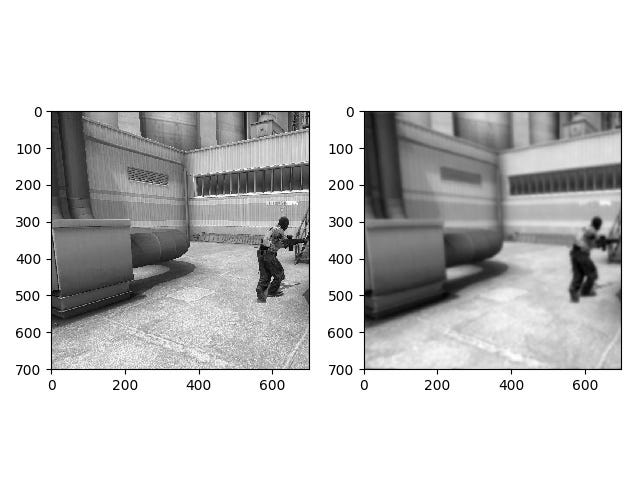You can see that the filtered one (right) is blurred. This is because higher frequency components (details) are removed and only lower frequency components (rough shape) remain unchanged.

# Implementation in Python

You can use whatever image you like. We first pick up one color channel and crop it generating a gray-scale square image. Then a Hamming window is defined with a parameter ‘r’. This value specified how small the window is. The larger this value is, the more blur the filtered image is.

```import cv2
import numpy as npimg_path = './test_image.jpg'
img = cv2.imread(img_path)[:,:,0] # gray-scale image
img = img[:700, :700] # crop to 700 x 700 r = 50 # how narrower the window is
ham = np.hamming(700)[:,None] # 1D hamming
ham2d = np.sqrt(np.dot(ham, ham.T)) ** r # expand to 2D hamming
```

Then we apply Fourier Transform on the image with opencv. The output of this function is a numpy array of two channels, which are imaginary and real parts, respectively. The Hamming window (filter) is applied just by multiplying it by the complex version of the transformed image.

```f = cv2.dft(img.astype(np.float32), flags=cv2.DFT_COMPLEX_OUTPUT)
f_shifted = np.fft.fftshift(f)
f_complex = f_shifted[:,:,0]*1j + f_shifted[:,:,1]
f_filtered = ham2d * f_complex
```

Don’t forget to cast the data type of the image to 32-bit float, otherwise, the function does not work.
Since the center of the image does not coincide with the origin, we have to handle this problem with `np.fft.fftshift()` function. What this function does is just divide an image into four small images, and then rearrange them such that it becomes symmetric about the center.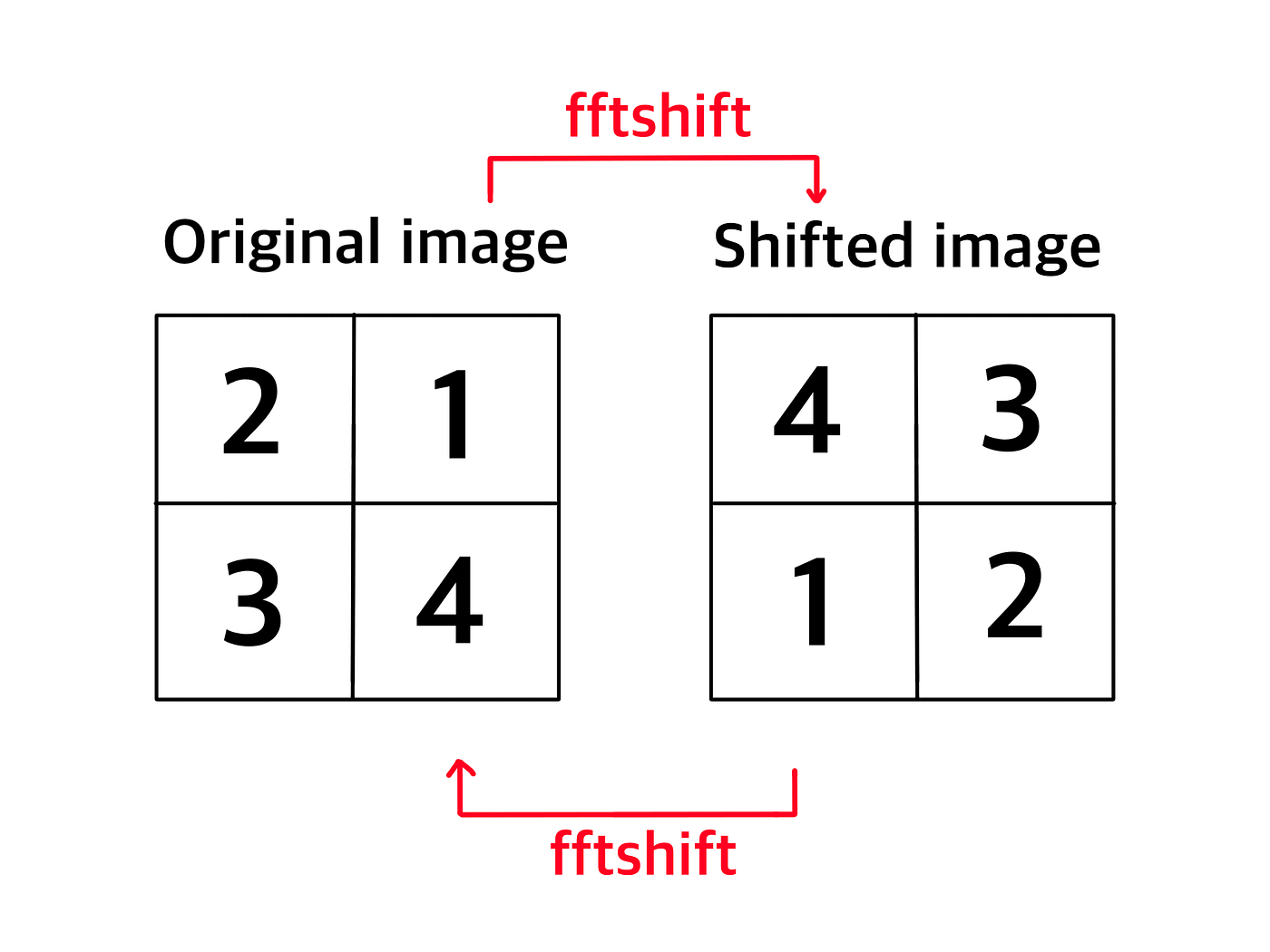If we apply fftshift() against a shifted image, then the original image is restored.

If we do not apply shifting, the transformed image looks like the following.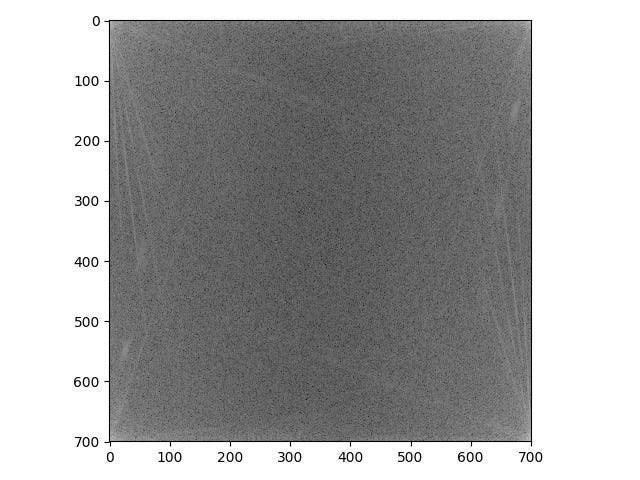This is not a Fourier Transformed image we usually see, in that it does not look like an asteroid or a star.

Then we apply Inverse Fourier Transform on `f_filterd` and expand the result such that all values are between 0 and 255. Do not forget to restore the shifting again with `fftshift()` function, otherwise, the resulting image would be a blurred, bud-shifted image about the center.

```f_filtered_shifted = np.fft.fftshift(f_filtered)
inv_img = np.fft.ifft2(f_filtered_shifted) # inverse F.T.
filtered_img = np.abs(inv_img)
filtered_img -= filtered_img.min()
filtered_img = filtered_img*255 / filtered_img.max()
filtered_img = filtered_img.astype(np.uint8)
```

References:

https://homepages.inf.ed.ac.uk/rbf/HIPR2/fourier.htm

https://www.tutorialspoint.com/dip/fourier_series_and_transform.htm

https://www.cs.unm.edu/~brayer/vision/fourier.html

http://www.imagemagick.org/Usage/fourier/

https://betterexplained.com/articles/an-interactive-guide-to-the-fourier-transform/##### Amir Masoud Sefidian
Machine Learning Engineer